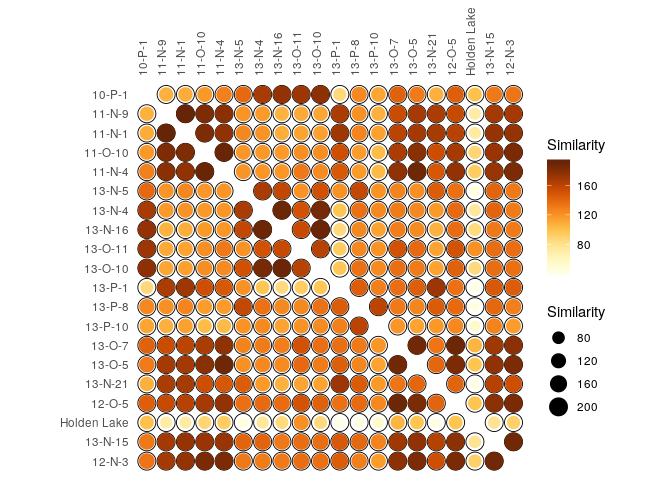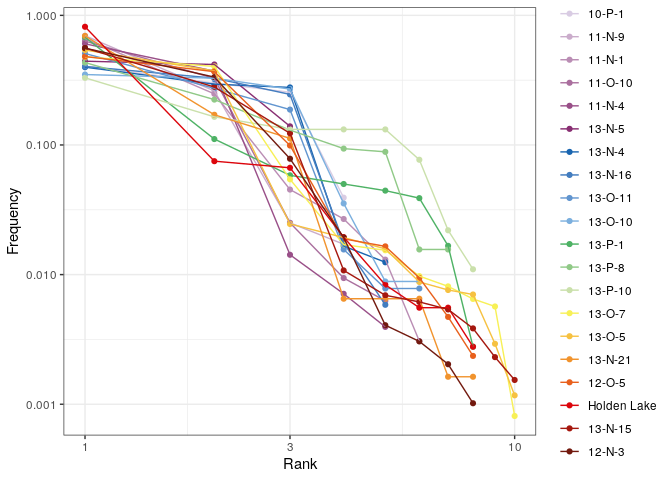2. Diversity Measures

2019-09-20

library(tabula)
library(magrittr)

Thereafter, we denote by:

• $$S$$ the total number of taxa recorded,
• $$i$$ the rank of the taxon
• $$n_i$$ the number of individuals in the $$i$$-th taxon,
• $$N = \sum n_i$$ the total number of individuals,
• $$p_i$$ the relative proportion of the $$i$$-th taxon in the population,
• $$s_k$$ the number of taxa with $$k$$ individuals,
• $$q_i$$ the incidence of the $$i$$-th taxon.

1$$\alpha$$-diversity

1.1 Richness

1.1.1 Definitions

The number of different taxa, provides an instantly comprehensible expression of diversity. While the number of taxa within a sample is easy to ascertain, as a term, it makes little sense: some taxa may not have been seen, or there may not be a fixed number of taxa (e.g. in an open system; Peet 1974). As an alternative, richness ($$S$$) can be used for the concept of taxa number (McIntosh 1967).

1.1.1.1 Margalef index (Margalef 1958)

$S_{Mg} = \frac{S - 1}{\ln N}$

1.1.1.2 Menhinick index (Menhinick 1964)

$S_{Mn} = \frac{S}{\sqrt{N}}$

1.1.1.3 Chao1 estimator (Chao 1984)

$\hat{S}_{Chao1} = \begin{cases} S + \frac{N - 1}{N} \frac{s_1^2}{2 s_2} & s_2 > 0 \\ S + \frac{N - 1}{N} \frac{s_1 (s_1 - 1)}{2} & s_2 = 0 \end{cases}$

In the special case of homogeneous case, a bias-corrected estimator is:

$\hat{S}_{Chao1-bc} = S + \frac{N - 1}{N} \frac{s_1 (s_1 - 1)}{2 s_2 + 1}$

Improved Chao1 estimator (makes use of the additional information of tripletons and quadrupletons; Chiu et al. 2014) :

$\hat{S}_{iChao1} = \hat{S}_{Chao1} + \frac{N - 3}{4 N} \frac{s_3}{s_4} \times \max\left(s_1 - \frac{N - 3}{N - 1} \frac{s_2 s_3}{2 s_4} , 0\right)$

1.1.1.4 Abundance-based Coverage Estimator (Chao and Lee 1992)

$\hat{S}_{ACE} = \hat{S}_{abun} + \frac{\hat{S}_{rare}}{\hat{C}_{rare}} + \frac{s_1}{\hat{C}_{rare}} \times \hat{\gamma}^2_{rare}$

Where $$\hat{S}_{rare} = \sum_{i = 1}^{k} s_i$$ is the number of rare taxa, $$\hat{S}_{abun} = \sum_{i > k}^{N} s_i$$ is the number of abundant taxa (for a given cut-off value $$k$$), $$\hat{C}_{rare} = 1 - \frac{s_1}{N_{rare}}$$ is the Turing’s coverage estimate and:

$\hat{\gamma}^2_{rare} = \max\left[\frac{\hat{S}_{rare}}{\hat{C}_{rare}} \frac{\sum_{i = 1}^{k} i(i - 1)s_i}{\left(\sum_{i = 1}^{k} is_i\right)\left(\sum_{i = 1}^{k} is_i - 1\right)} - 1, 0\right]$

1.1.1.5 Chao2 estimator (Chao 1987)

For replicated incidence data (i.e. a $$m \times p$$ logical matrix), the Chao2 estimator is:

$\hat{S}_{Chao2} = \begin{cases} S + \frac{m - 1}{m} \frac{q_1^2}{2 q_2} & q_2 > 0 \\ S + \frac{m - 1}{m} \frac{q_1 (q_1 - 1)}{2} & q_2 = 0 \end{cases}$

Improved Chao2 estimator (Chiu et al. 2014):

$\hat{S}_{iChao2} = \hat{S}_{Chao2} + \frac{m - 3}{4 m} \frac{q_3}{q_4} \times \max\left(q_1 - \frac{m - 3}{m - 1} \frac{q_2 q_3}{2 q_4} , 0\right)$

1.1.1.6 Incidence-based Coverage Estimator (Chao and Chiu 2016)

$\hat{S}_{ICE} = \hat{S}_{freq} + \frac{\hat{S}_{infreq}}{\hat{C}_{infreq}} + \frac{q_1}{\hat{C}_{infreq}} \times \hat{\gamma}^2_{infreq}$

Where $$\hat{S}_{infreq} = \sum_{i = 1}^{k} q_i$$ is the number of infrequent taxa, $$\hat{S}_{freq} = \sum_{i > k}^{N} q_i$$ is the number of frequent taxa (for a given cut-off value $$k$$), $$\hat{C}_{infreq} = 1 - \frac{Q_1}{\sum_{i = 1}^{k} iq_i}$$ is the Turing’s coverage estimate and:

$\hat{\gamma}^2_{infreq} = \max\left[\frac{\hat{S}_{infreq}}{\hat{C}_{infreq}} \frac{m_{infreq}}{m_{infreq} - 1} \frac{\sum_{i = 1}^{k} i(i - 1)q_i}{\left(\sum_{i = 1}^{k} iq_i\right)\left(\sum_{i = 1}^{k} iq_i - 1\right)} - 1, 0\right]$

Where $$m_{infreq}$$ is the number of sampling units that include at least one infrequent species.

1.2 Rarefaction

It is not always possible to ensure that all sample sizes are equal and the number of different taxa increases with sample size and sampling effort (Magurran 1988). Then, rarefaction ($$E(S)$$) is the number of taxa expected if all samples were of a standard size $$n$$ (i.e. taxa per fixed number of individuals). Rarefaction assumes that imbalances between taxa are due to sampling and not to differences in actual abundances.

Hurlbert (1971) unbiased estimate of Sander (1968) rarefaction:

$E(S) = \sum_{i = 1}^{S} 1 - \frac{{N - N_i} \choose n}{N \choose n}$ Where $$n$$ is the sub-sample size.

1.3 Diversity and evenness

1.3.1 Definitions

Diversity measurement assumes that all individuals in a specific taxa are equivalent and that all types are equally different from each other (Peet 1974). A measure of diversity can be achieved by using indices built on the relative abundance of taxa. These indices (sometimes referred to as non-parametric indices) benefit from not making assumptions about the underlying distribution of taxa abundance: they only take relative abundances of the species that are present and species richness into account. Peet (1974) refers to them as indices of heterogeneity ($$H$$).

Diversity indices focus on one aspect of the taxa abundance and emphasize either richness (weighting towards uncommon taxa) or dominance (weighting towards abundant taxa; Magurran 1988).

Evenness ($$E$$) is a measure of how evenly individuals are distributed across the sample.

1.3.1.1 Information theory index

1.3.1.1.1 Shannon-Wiener diversity index

The Shannon-Wiener index (Shannon 1948) assumes that individuals are randomly sampled from an infinite population and that all taxa are represented in the sample (it does not reflect the sample size). The main source of error arises from the failure to include all taxa in the sample: this error increases as the proportion of species discovered in the sample declines (Peet 1974; Magurran 1988). The maximum likelihood estimator (MLE) is used for the relative abundance, this is known to be negatively biased by sample size.

Diversity:

$H' = - \sum_{i = 1}^{S} p_i \ln p_i$

Evenness:

$E = \frac{H'}{H'_{max}} = \frac{H'}{\ln S} = - \sum_{i = 1}^{S} p_i \log_S p_i$

When $$p_i$$ is unknown in the population, an estimate is given by $$\hat{p}_i =\frac{n_i}{N}$$ (maximum likelihood estimator - MLE). As the use of $$\hat{p}_i$$ results in a biased estimate, Hutcheson (1970) and Bowman et al. (1971) suggest the use of:

$\hat{H}' = - \sum_{i = 1}^{S} \hat{p}_i \ln \hat{p}_i - \frac{S - 1}{N} + \frac{1 - \sum_{i = 1}^{S} \hat{p}_i^{-1}}{12N^2} + \frac{\sum_{i = 1}^{S} (\hat{p}_i^{-1} - \hat{p}_i^{-2})}{12N^3} + \cdots$

This error is rarely significant (Peet 1974), so the unbiased form is not implemented here (for now).

1.3.1.1.2 Brillouin diversity index

The Brillouin index (Brillouin 1956) describes a known collection: it does not assume random sampling in an infinite population. Pielou (1975) and Laxton (1978) argues for the use of the Brillouin index in all circumstances, especially in preference to the Shannon index.

Diversity:

$HB = \frac{\ln (N!) - \sum_{i = 1}^{S} \ln (n_i!)}{N}$

Evenness:

$E = \frac{HB}{HB_{max}}$

with:

$HB_{max} = \frac{1}{N} \ln \frac{N!}{\left( \lfloor \frac{N}{S} \rfloor! \right)^{S - r} \left[ \left( \lfloor \frac{N}{S} \rfloor + 1 \right)! \right]^{r}}$

where: $$r = N - S \lfloor \frac{N}{S} \rfloor$$.

1.3.1.2 Dominance index

The following methods return a dominance index, not the reciprocal or inverse form usually adopted, so that an increase in the value of the index accompanies a decrease in diversity.

1.3.1.2.1 Simpson index

The Simpson index (Simpson 1949) expresses the probability that two individuals randomly picked from a finite sample belong to two different types. It can be interpreted as the weighted mean of the proportional abundances. This metric is a true probability value, it ranges from $$0$$ (perfectly uneven) to $$1$$ (perfectly even).

Dominance for an infinite sample:

$D = \sum_{i = 1}^{S} p_i^2$

Dominance for a finite sample:

$\lambda = \sum_{i = 1}^{S} \frac{n_i \left( n_i - 1 \right)}{N \left( N - 1 \right)}$

1.3.1.2.2 McIntosh index

The McIntosh index (McIntosh 1967) expresses the heterogeneity of a sample in geometric terms. It describes the sample as a point of a $$S$$-dimensional hypervolume and uses the Euclidean distance of this point from the origin.

Dominance:

$D = \frac{N - U}{N - \sqrt{N}}$

Evenness:

$E = \frac{N - U}{N - \frac{N}{\sqrt{S}}}$

where $$U$$ is the distance of the sample from the origin in an $$S$$ dimensional hypervolume:

$U = \sqrt{\sum_{i = 1}^{S} n_i^2}$

1.3.1.2.3 Berger-Parker index

The Berger-Parker index (Berger and Parker 1970) expresses the proportional importance of the most abundant type. This metric is highly biased by sample size and richness, moreover it does not make use of all the information available from sample.

Dominance:

$d = \frac{n_{max}}{N}$

2$$\beta$$-diversity

2.1 Turnover

2.1.1 Definitions

The following methods can be used to ascertain the degree of turnover in taxa composition along a gradient on qualitative (presence/absence) data. This assumes that the order of the matrix rows (from 1 to $$m$$) follows the progression along the gradient/transect.

We denote the $$m \times p$$ incidence matrix by $$X = \left[ x_{ij} \right] ~\forall i \in \left[ 1,m \right], j \in \left[ 1,p \right]$$ and the $$p \times p$$ corresponding co-occurrence matrix by $$Y = \left[ y_{ij} \right] ~\forall i,j \in \left[ 1,p \right]$$, with row and column sums:

\begin{align} x_{i \cdot} = \sum_{j = 1}^{p} x_{ij} && x_{\cdot j} = \sum_{i = 1}^{m} x_{ij} && x_{\cdot \cdot} = \sum_{j = 1}^{p} \sum_{i = 1}^{m} x_{ij} && \forall x_{ij} \in \lbrace 0,1 \rbrace \\ y_{i \cdot} = \sum_{j \geqslant i}^{p} y_{ij} && y_{\cdot j} = \sum_{i \leqslant j}^{p} y_{ij} && y_{\cdot \cdot} = \sum_{i = 1}^{p} \sum_{j \geqslant i}^{p} y_{ij} && \forall y_{ij} \in \lbrace 0,1 \rbrace \end{align}

2.1.1.1 Whittaker measure (Whittaker 1960)

$\beta_W = \frac{S}{\alpha} - 1$

where $$\alpha$$ is the mean sample diversity: $$\alpha = \frac{x_{\cdot \cdot}}{m}$$.

2.1.1.2 Cody measure (Cody 1975)

$\beta_C = \frac{g(H) + l(H)}{2} - 1$

where $$g(H)$$ is the number of taxa gained along the transect and $$l(H)$$ the number of taxa lost.

2.1.1.3 Routledge measures (Routledge 1977)

\begin{align} \beta_R &= \frac{S^2}{2 y_{\cdot \cdot} + S} - 1 \\ \beta_I &= \log x_{\cdot \cdot} - \frac{\sum_{j = 1}^{p} x_{\cdot j} \log x_{\cdot j}}{x_{\cdot \cdot}} - \frac{\sum_{i = 1}^{m} x_{i \cdot} \log x_{i \cdot}}{x_{\cdot \cdot}} \\ \beta_E &= \exp(\beta_I) - 1 \end{align}

2.1.1.4 Wilson and Shmida measure (Wilson and Shmida 1984)

$\beta_T = \frac{g(H) + l(H)}{2\alpha}$

where $$g(H)$$ is the number of taxa gained along the transect, $$l(H)$$ the number of taxa lost and $$\alpha$$ the mean sample diversity, $$\alpha = \frac{x_{\cdot \cdot}}{m}$$.

2.2 Similarity

2.2.1 Definitions

Similarity between two samples $$a$$ and $$b$$ or between two types $$x$$ and $$y$$ can be measured as follow.

These indices provide a scale of similarity from $$0$$-$$1$$ where $$1$$ is perfect similarity and $$0$$ is no similarity, with the exception of the Brainerd-Robinson index which is scaled between $$0$$ and $$200$$.

$$a_j$$ and $$b_j$$ denote the number of individuals in the $$j$$-th type/taxon, $$j \in \left[ 1,n \right]$$. $$o_j$$ denotes the number of type/taxon common to both sample/case: $$o_j = \sum_{k = 1}^{n} a_k \cap b_k$$.

$$x_i$$ and $$y_i$$ denote the number of individuals in the $$i$$-th sample/case, $$i \in \left[ 1,m \right]$$. $$o_i$$ denotes the number of sample/case common to both type/taxon: $$o_i = \sum_{k = 1}^{m} x_k \cap y_k$$.

2.2.1.1 Qualitative indices

2.2.1.1.1 Jaccard index

$C_J = \frac{o_j}{S_a + S_b - o_j}$

2.2.1.1.2 Sorenson index

$C_S = \frac{2 \times o_j}{S_a + S_b}$

2.2.1.2 Quantitative index

2.2.1.2.1 Brainerd-Robinson index (Brainerd 1951; Robinson 1951)

$C_{BR} = 200 - \sum_{j = 1}^{S} \left| \frac{a_j \times 100}{\sum_{j = 1}^{S} a_j} - \frac{b_j \times 100}{\sum_{j = 1}^{S} b_j} \right|$

2.2.1.2.2 Sorenson index

Bray and Curtis (1957) modified version of Sorenson’s index:

$C_N = \frac{2 \sum_{j = 1}^{S} \min(a_j, b_j)}{N_a + N_b}$

2.2.1.2.3 Morisita-Horn index

$C_{MH} = \frac{2 \sum_{j = 1}^{S} a_j \times b_j}{(\frac{\sum_{j = 1}^{S} a_j^2}{N_a^2} + \frac{\sum_{j = 1}^{S} b_j^2}{N_b^2}) \times N_a \times N_b}$

2.2.1.2.4 Binomial co-occurrence (Kintigh 2006)

$C_{Bi} = \frac{o_i - N \times p}{\sqrt{N \times p \times (1 - p)}}$

2.2.2 Usage3 Abundance models

Ranks vs abundance plot can be used for abundance models (model fitting will be implemented in a future release):

mississippi %>%
as_count() %>%
plot_rank(log = "xy", facet = FALSE) +
ggplot2::theme_bw() +
khroma::scale_color_discreterainbow()